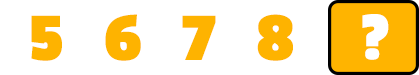# What is the Next Number?

Description: Count forward beginning from a given number within the known sequence.

Tags: Kindergarten, Counting, Counting to 10, CCSS.MATH.CONTENT.K.CC.A.2, Kindergarten Math Problems

Question 1 (Multiple Choice, 10 points)

What is the next number?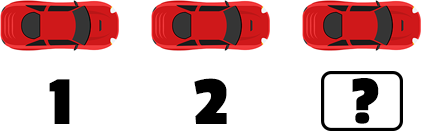Question 2 (Multiple Choice, 10 points)

What is the next number?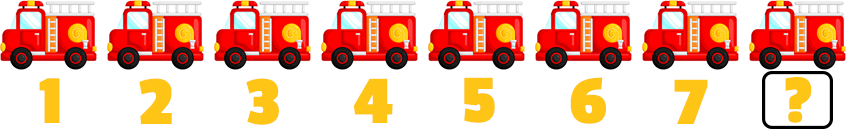Question 3 (Short Answer, 10 points)

What is the next number?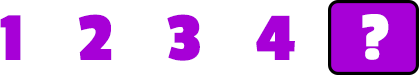Question 4 (Multiple Choice, 10 points)

What is the next number?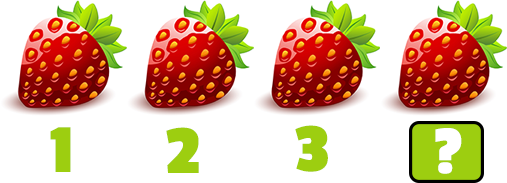Question 5 (Short Answer, 10 points)

What is the next number?